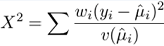# 1.1 - 8.10 - Pearson’s Chi-squared Statistic - Teradata Vantage

## Teradata Vantage™ - Machine Learning Engine Analytic Function Reference

Product
Release Number
1.1
8.10
Published
October 2019
Content Type
Programming Reference
Publication ID
B700-4003-079K
Language
English (United States)

The deviance generalizes the sum of squared errors. Another generalization of sum of squared errors is Pearson’s chi-squared statistic. Given a generalized linear model with responses yi, weights wi, fitted means μi, variance function v(μ) and dispersion φ = 1, the Pearson goodness-of-fit statistic isIf the fitted model is correct and the observations yi are approximately normal, X2 is approximately distributed as X2on the residual degrees of freedom for the model. Both the deviance and the generalized Pearson X2 have exact X2 distributions for Normal-theory linear models (assuming of course that the model is true), and asymptotic results are available for the other distributions. The deviance has a general advantage as a measure of discrepancy in that it is additive for nested sets of models if maximum-likelihood estimates are used, whereas X2 in general is not. However, X2 may sometimes be preferred because of its more direct interpretation.

The GLM function computes the Pearson’s goodness of fit.